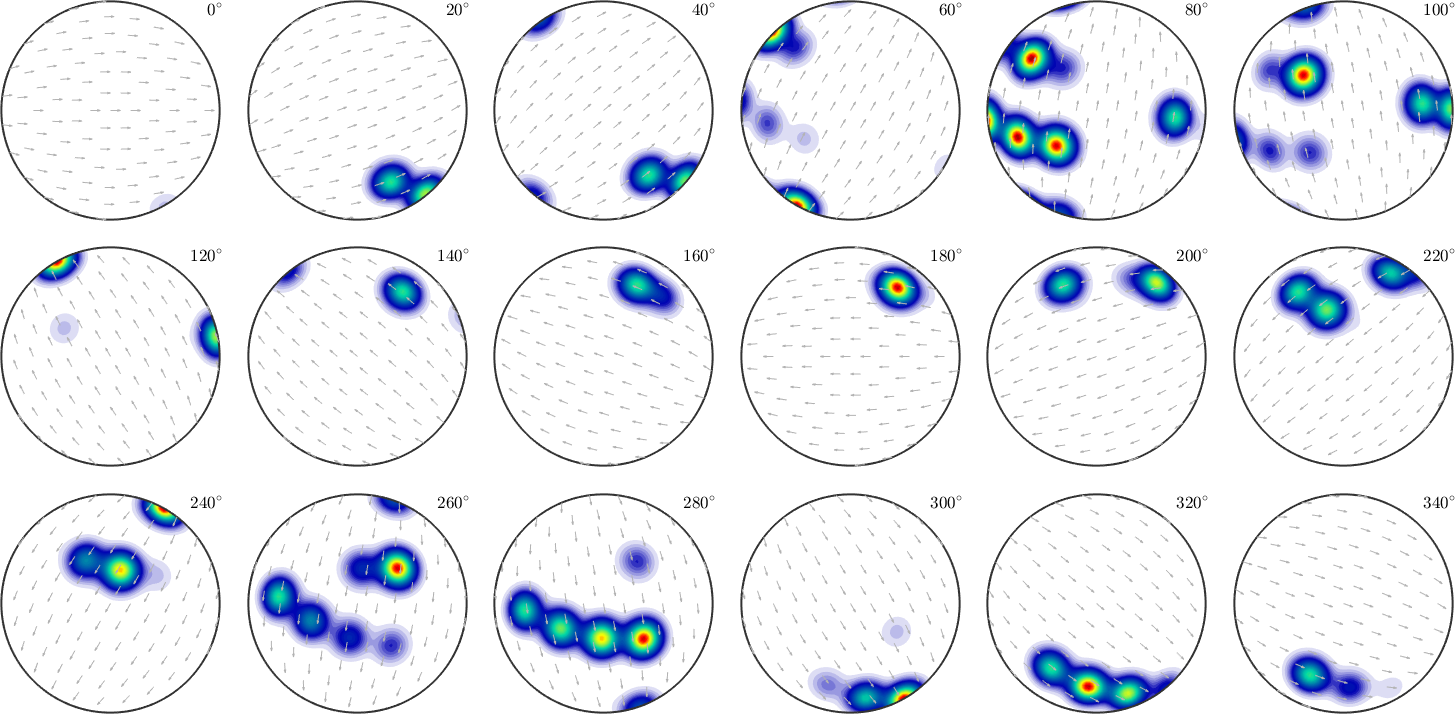Sigma Sections edit page

Although $$\varphi_2$$ sections are most common to represent orientation distribution functions they heavily suffer from geometrical distortions of the orientation space. Lets illustrate this at a simple example. The following $$\varphi_2$$ sections represent a hexagonal ODF composod from several unimodal components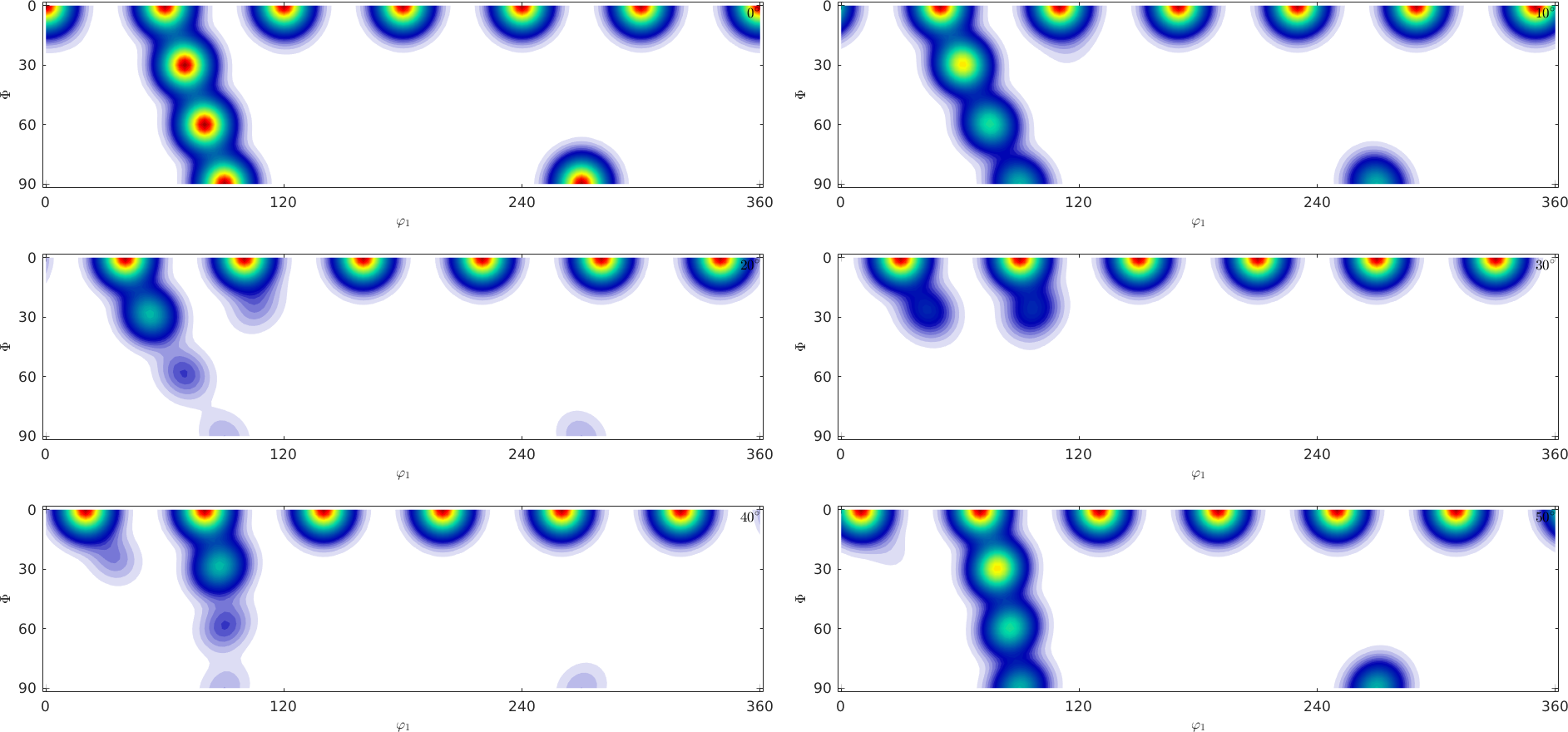Try to answer the following questions:

1. What is the number of components the ODF is composed of?
2. What would the c-axis pole figure look like?
3. What would the a-axis pole figure look like?

Most people find it difficult to find the correct answer by looking at $$\varphi_2$$ sections, while it is much more easy by looking at $$\sigma$$-sections.

Lets consider an arbitrary orientation given by its Euler angles $$(\varphi_1, \Phi, \varphi_2)$$. Then its position in the c-axis pole figure is given by the polar coordinates $$(\Phi,\varphi_1)$$, i.e. it depends only on the first two Euler angles. The third Euler angle $$\varphi_2$$ controls the rotation of the crystal around this new c-axis. This rotation around the new c-axis can be described by the angle between the a-axis with respect to some fixed reference direction. In the case of hexagonal symmetry this angle may vary between $$0$$ and $$60$$ degree.

The idea of sigma sections is to make a reasonable choice of this reference direction.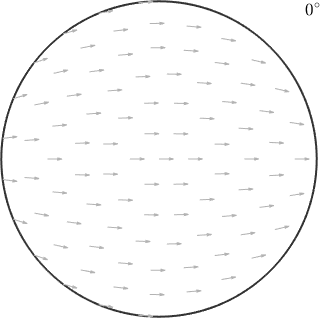In the above plot each pixel correspondes to a unique orientation. Which is specified by the position of the c-axis beeing in the position of the pixel and the a-axis beeing aligned with the small arrow at this position. As an example lets consider the orientation

that maps the c-axis parallel to the z-direction and the a-axis parallel to the x-direction and the orientation

that maps the c-axis parallel to the x-axis and the a-axis parallel to the z-axis.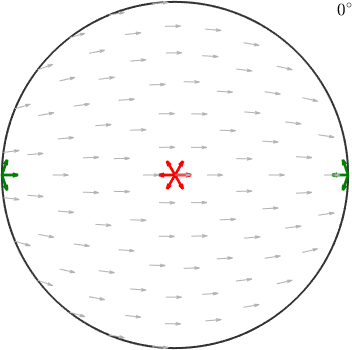Accordingly, the first orientations appears right in the center while the second one appears at the position of the x-axis. The red and green arrows indicate the directions of the a-axes and align perfectly with the small background arrows.

In order to visualize orientations with different a-axis alignments we need to additional sections with rotated small arrows.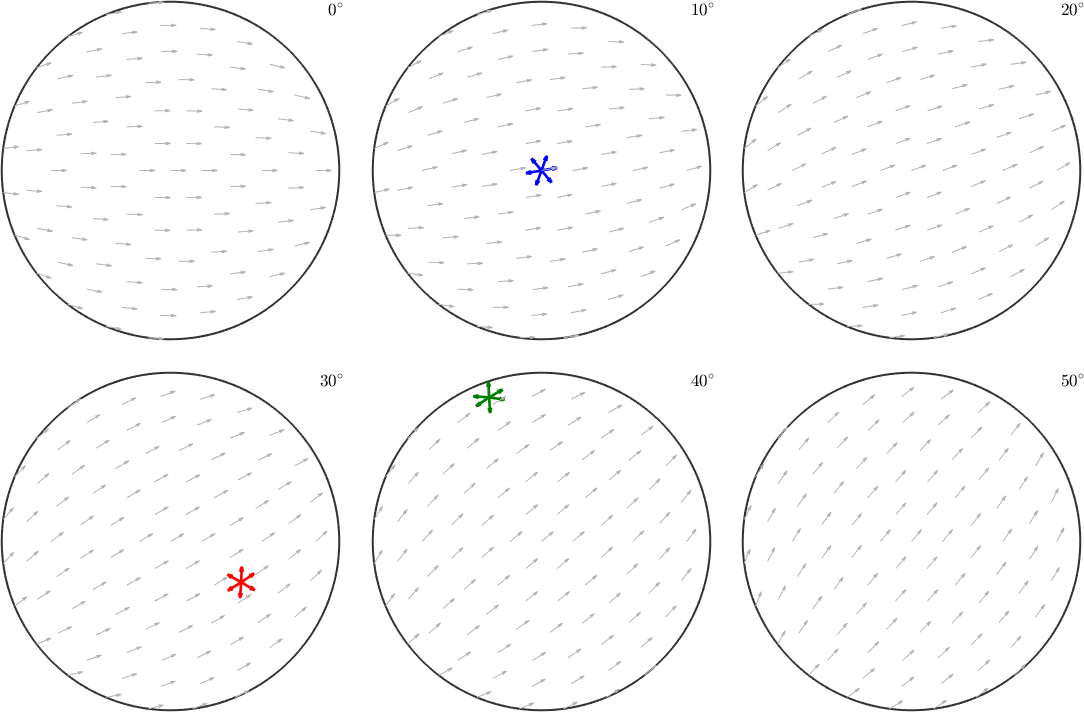Note how the a-axes of the three orientations align with the small background arrows. Instead of the a-axes we may also visualize the crystal orientations directly within these sigma sections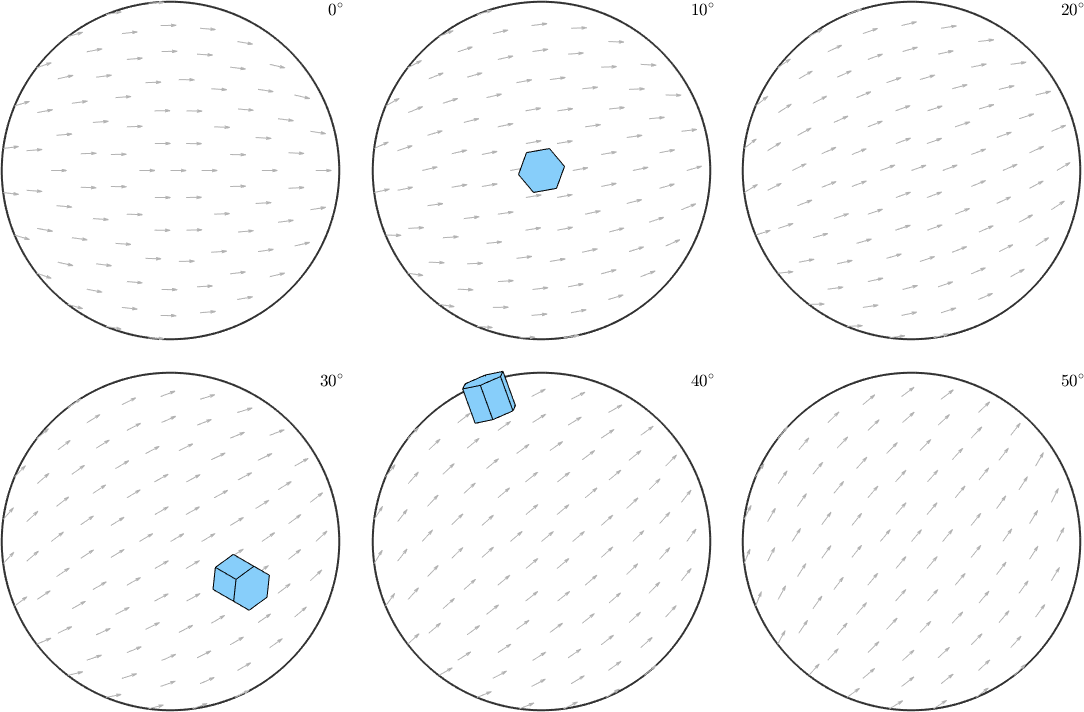Lets come back to our initial secret ODF and visualize it in sigma sections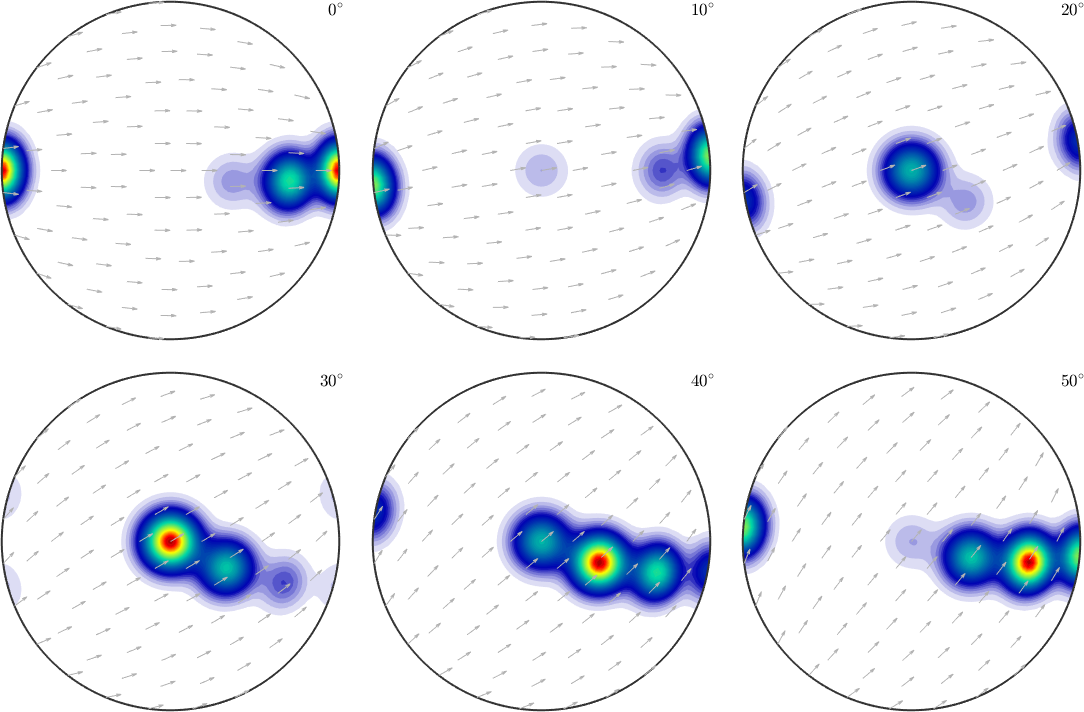First of all we observe clearly 4 distinct components with the first one having its maximum for the c-axis parallel to the z-axis and the a-axis parallel to the y-axis. With the other three components the c-axis rotates toward the x-axis while the a-axis rotates towards the z-axis. Hence, we would expect in the c-axis a girdle from $$z$$ to $$x$$ and in the a-axis pole figure ...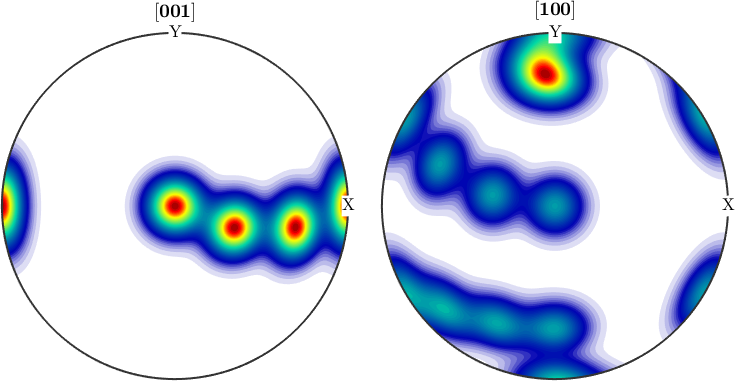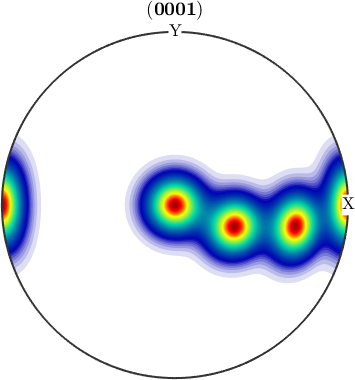We observe three spots. Two in the center and one at 100. When splitting the pole figure, i.e. plotting the odf as sigma sections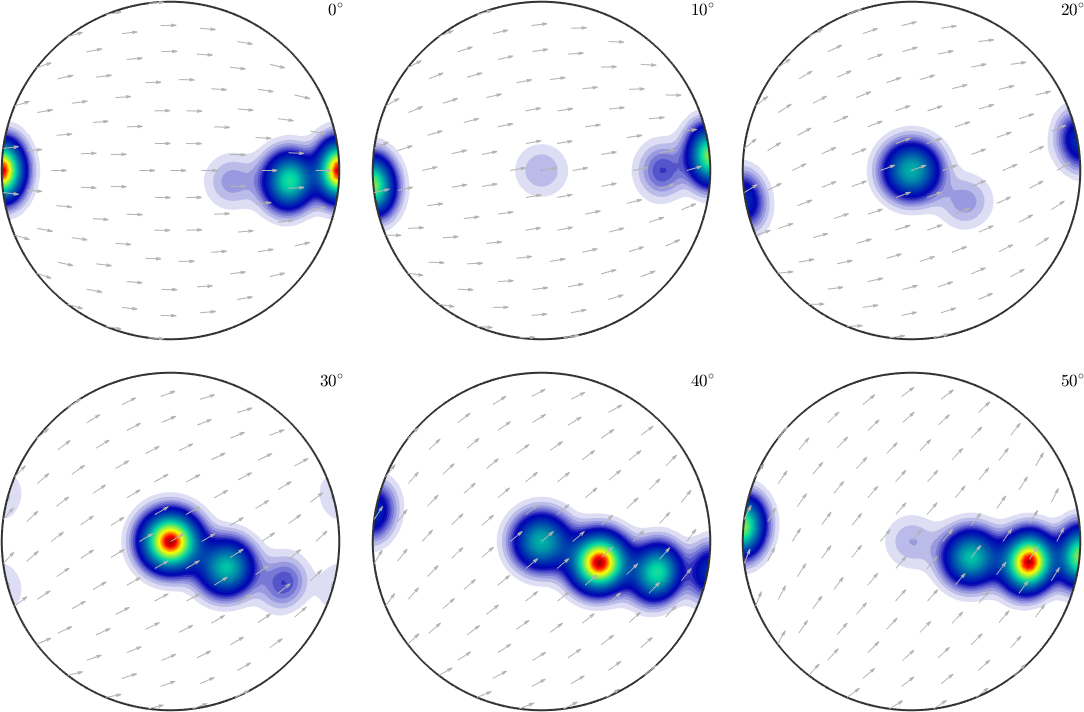we can clearly distinguish the two spots in the middle indicating two radial symmetric portions. On the other hand the spots at 001 appear in every section indicating a fibre at position (100). Knowing that sigma sections are nothing else then the splitted 001 pole figure they are much more simple to interpret then usual phi2 sections.

## Customizations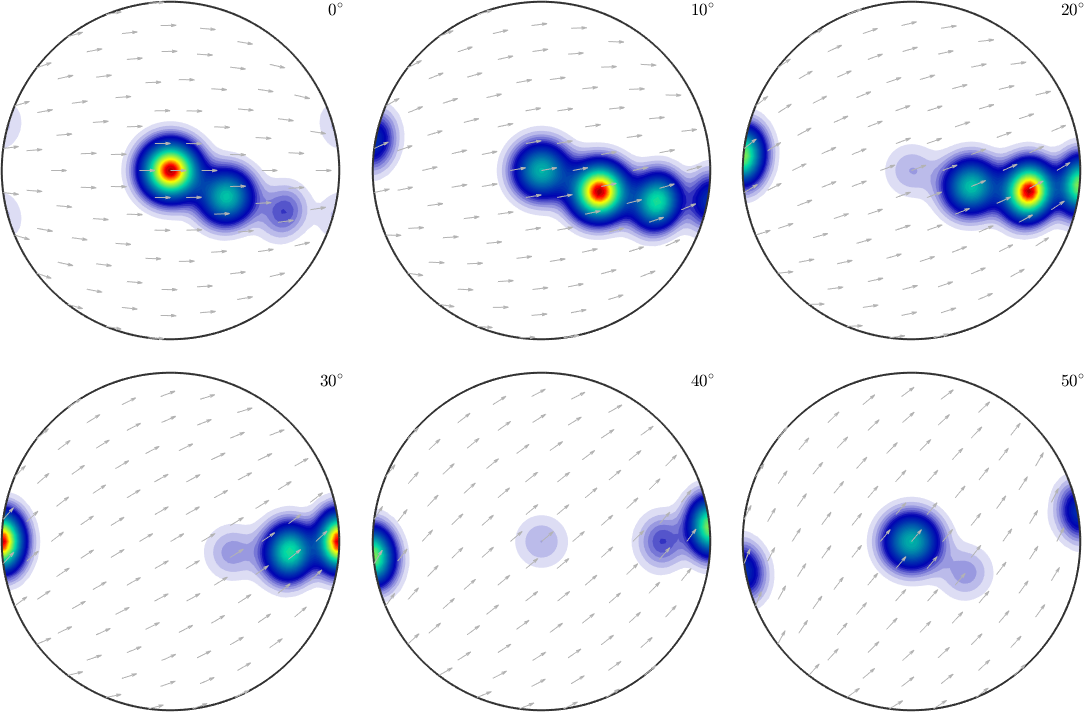We may also change the pole figure we would like to split into sections.